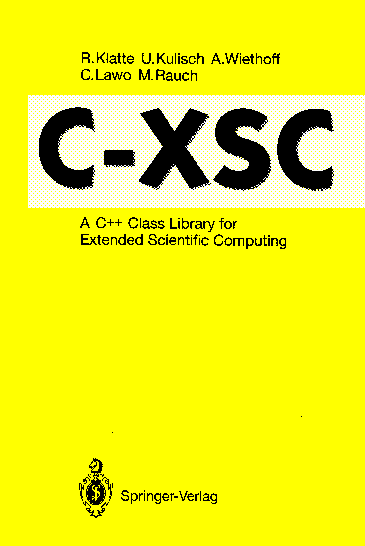C-XSC# C-XSC

## - A C++ Class Library for Extended Scientific Computing -

Springer-Verlag, Heidelberg (ISBN 3-540-56328-8), New York (ISBN 0-387-56328-8), 1993.

## Summary

C-XSC is a tool for the development of numerical algorithms delivering highly accurate and automatically verified results. It provides a large number of predefined numerical data types and operators. These types are implemented as C++ classes. Thus, C-XSC allows high-level programming of numerical applications in C and C++.

The most important features of C-XSC are:

• Real, complex, interval and complex interval arithmetic
• Standard functions of high accuracy
• Predefined arithmetic operators with maximum accuracy for real, complex, interval and complex interval vectors and matrices
• Dynamic vectors and matrices
• Subarrays of vectors and matrices
• Multiple- precision arithmetic and standard functions
• Dotprecision data types
• Rounding control for I/O data
• Library of problem solving routines with automatic result verification
• Thus, C-XSC makes the computer more powerful concerning the arithmetic, C-XSC is immediately usable by C programmers, easy to learn, user-extendable, and may also be combined with other tools.

The book can be used as textbook and as a reference manual. It consists of an introduction to advanced computer arithmetic, a chapter describing the programming languages C and C++, the major chapter "C-XSC Reference", sample programs, and indices.